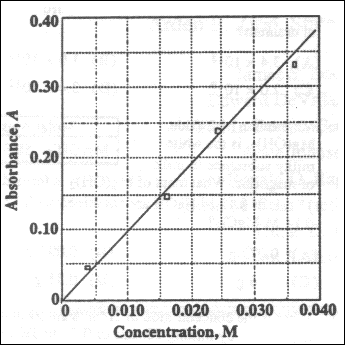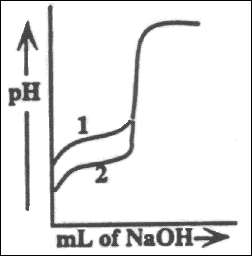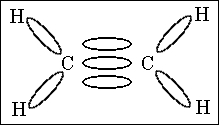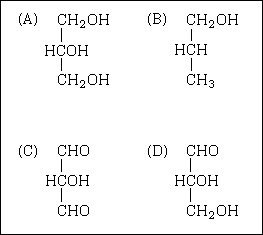MainTutorialsOrganic ChemistryPractice TestsOnline QuizzesReference ToolsU.S. National Chemistry Olympiad: 1997 National Test

1. The formula of sodium tungstate is Na2WO4 and that of lead phosphate is Pb3(PO4)2. What is the formula for lead tungstate?

(A) PbWO4
(B) Pb2(WO4)3
(C) Pb3(WO4)2
(D) Pb3(WO4)4

2. A metal from period 4 is added to water and a vigorous reaction takes place with the evolution of a gas. Which statements are correct?

1. Oxygen is evolved.
2. Hydrogen is evolved.
3. The resulting solution is acidic.
4. The resulting solution is basic.

(A) 1 and 3 only
(B) 2 and 3 only
(C) 2 and 4 only
(D) 1 and 4 only

3. 30.mL of 0.10 Ba(OH)2(aq) is added to 30. mL of 0.10 M H2SO4(aq) and the solution is stirred quickly. The rise in temperature, DT1, is measured. The experiments is repeated using 90.mL of each solution and the rise in temperature, DT1, is measured. What is the relationship between DT1 and DT2?

(A) DT2 is equal to DT1
(B) DT2 is three times as large as DT1
(C) DT2 is six times as large as DT1
(D) DT2 is one-third as large as DT1

4. An aqueous solution is 0.1 M in each of these ions.

1. Cu2+
2. Hg2+
3. Pb2+

Which ion(s) will precipitate if 0.1 M HCI is added?

(A) 2 only
(B) 3 only
(C) 2 and 3 only
(D) 1,2, and 3

5. The age of a wooden artifact was determined by carbon-14 dating. Three different measurements gave values of 6925, 4815, and 5485 years. Which is the best value to report for the age of this artifact?

(A) 5700 years
(B) 5740 years
(C) 5741 years
(D) 6000 years

6. What is the molality, m, of methanol in a solution prepared by dissolving 160 g of methanol, CH3OH, in 200.0 g of water?

(A) 1.0 m
(B) 5.0 m
(C) 10.0 m
(D) 25.0 m

7. What is the coefficient for oxygen when this equation is balanced?

Sb2S3(s) + O2(g) ---> Sb2O3(s) + SO2(g)

(A) 9
(B) 7
(C) 5
(D) 4

8. Flourine reacts with uranium hexaflouride, UF6, as represented by this equation.

U(s) + 3F2(g) ---> UF6(g)

How many flourine molecules are required to produce 2.0 mg of uranium hexaflouride, UF6, from an excess of uranium? The molar mass of UF6 is 352.0 g mol�1

(A) 3.4 x 1018
(B) 1.0 x 1019
(C) 2.0 x 1019
(D) 3.4 x 1021

9. Magnesium hydroxide, Mg(OH)2, is the white milky substance in milk of magnesia. What mass of Mg(OH)2 is formed when 15mL of 0.18 M NaOH are combined with 12mL of 0.14 M MgCl2? The molar mass of Mg(OH)2 is 58.3 g mol�1

(A) 0.079 g
(B) 0.097 g
(C) 0.16 g
(D) 0.31 g

10. What is the concentration of H+ in a solution that is prepared by mixing 50.0 mL of 0.50 M HCl with 200.0 mL of 0.25 M HCl?

(A) 0.30 M
(B) 0.35 M
(C) 0.40 M
(D) 0.45 M

11. Which concentrations can be calculated if the mole fraction and density of an aqueous solution of HCl are known?

1. molality
2. molarity
3. percent by mass

(A) 1 only
(B) 3 only
(C) 1 and 2 only
(D) 1, 2, and 3

12. The second law of thermodynamics states that the

(A) energy of the universe is increasing.
(B) energy of the universe is constant.
(C) entropy of the universe is increasing
(D) entropy of the universe is constant.

13. Which substance is expected to have the greatest lattice enthalpy?

(A) LiF
(B) LiI
(C) CsF
(D) CsI

14. When these substances are arranged in order of increasing boiling point (lowest boiling point first), what is the correct order?

(A) NH3 < PH3 < AsH3
(B) AsH3 < PH3 < NH3
(C) PH3 < AsH3 < NH3
(D) AsH3 < NH3 < PH315. The concentration of copper(II) ions in an unknown solution is determined by comparing the absorbance of a diluted sample of the unknown with the Beer's Law Plot at a constant wave length for several standard solutions. When a 2.0 mL sample of the unknown copper(II) ion solution is diluted to 5.0 mL, its absorbance is 0.29. What is the concentration of copper(II) ions in the unknown?

(A) 0.0062 M
(B) 0.012 M
(C) 0.031 M
(D) 0.078 M

16. Which values can be obtained from the information represented by the vapor pressure curve of a liquid?

1. normal boiling point
2. normal freezing point
3. enthalpy of vaporization

(A) 1 only
(B) 1 and 2 only
(C) 1 and 3 only
(D) 1, 2, and 3

17. The enthalpy of fusion of ice is 6.02 kJ mol�1. The heat capacity of water is 4.18 J g�1 �C�1. What is the smallest number of ice cubes at O�C, each containing one mole of water, that are needed to cool 500. g of liquid water from 20�C to O�C?

(A) 1
(B) 7
(C) 14
(D) 125

18. As O2(l) is cooled at 1 atm pressure, it freezes to form Solid I at 54.5 K. At a lower temperature, Solid I rearranges to Solid II, which has a different crystal structure. Thermal measurements show that for the phase transition Solid I to Solid II, DH= -743.1 J mol�1 and DS = -17.0 J K�1 mol�1. At what temperature are solids I and II in equilibrium?

(A) 2.06 K
(B) 31.5 K
(C) 43.7 K
(D) 53.4 K19. What will happen to the state of a substance represented by this phase diagram when its temperature and pressure are changed from 60�C and 0.2 atm to 40�C and 1.0 atm?

(A) liquid to solid
(B) gas to solid
(C) solid to liquid
(D) solid to gas

20. All are state functions except

(A) pressure.
(B) heat.
(C) free energy.
(D) enthalpy.

21. Use the bond energies in the table to estimate DH for this reaction.

H2C=CH2 + Cl2 --> ClH2C-CH2Cl

 Bond Energies C - C 347 kJ mol�1 C = C 612 kJ mol�1 C - Cl 331 kJ mol�1 C - H 414 kJ mol�1 Cl - Cl 243 kJ mol�1

(A) DH = -684 kJ
(B) DH = -154 kJ
(C) DH = +89 kJ
(D) DH = +177 kJ

22. The free energy of formation of NO is 78 kJ mol�1 at the temperature of an automobile engine (1000 K). What is the equilibrium constant for this reaction at 1000 K?

(1/2) N2(g) + (1/2) O2(g) <===> NO(g)

(A) 8.4 x 10�5
(B) 7.1 x 10�9
(C) 4.2 x 10�10
(D) 1.7 x 10�19

23. For which reaction will DH= DE? Assume each reaction is carried out in an open container.

(A) 2CO(g) + O2(g) ---> 2CO2(g)
(B) H2(g) + Br2(g) ---> 2HBr(g)
(C) C(s) + 2H2O(g) ---> 2H2(g) + CO2(g)
(D) PCl5(g) ---> PCl3(g) + Cl2(g)

24. A certain zero-order reaction has k = 0.025 M s�1 for the disapperance of A. What will be the concentration of A after 15 seconds if the initial concentration is 0.50 M?

(A) 0.50 M
(B) 0.32 M
(C) 0.12 M
(D) 0.060 M

25. A first-order reaction has a half-life of 14.5 hours. What percentage of the reactant will remain after 24.0 hours?

(A) 18.3%
(B) 31.8%
(C) 45.5%
(D) 68.2%

26. The reaction of hydrogen and iodine monochloride is represented by this equation. P align=center>H2(g) + 2ICl(g) ---> 2HCl(g) + I2(g)

This reaction is first order in H2(g) and also first order in ICl(g). Which of these proposed mechanisms can be consistent with the given information about this reaction?

 Mechanism 1 H2(g) + 2ICl(g) ---> 2HCl(g) + I2(g) Mechanism 2 H2(g) + ICl(g) ---> HCl(g) + HI(g) slow HI(g) + ICl(g) ---> HCl(g) + I2(g) fast

(A) 1 only
(B) 2 only
(C) Both 1 and 2
(D) Neither 1 nor 2

27. The reaction of ozone with oxygen atoms in the presence of chlorine atoms can occur by the two-step process shown here with the rate constant for each step.

 O3(g) + Cl(g) ---> O2(g) + ClO(g) k1 = 5.2 x 109 L mol�1 s�1 ClO(g) + O(g) ---> Cl(g) + O2(g) k2 = 2.6 x 1010 L mol�1 s�1

Which of the values below is closest to the rate constant of the overall net reaction, given by this equation.

O3(g) + O(g) ---> 2O2(g)

(A) 5.2 x 109 L mol�1 s�1
(B) 2.6 x 1010 L mol�1 s�1
(C) 3.1 x 1010 L mol�1 s�1
(D) 1.4 x 1020 L mol�1 s�1

28. The first-order reaction:

2N2(g) ---> 2N2(g) + O2(g)

has a rate constant of 1.3 x 10�11 s�1 at 270�C and 4.5 x 10�10 s�1 at 350�C. What is the activation energy for this reaction?

(A) 15 kJ
(B) 30 kJ
(C) 68 kJ
(D) 120 kJ

29. A 0.010 M solution of maleic acid, a monoportic organic acid, is 14% ionized. What is Ka for maleic acid?

(A) 2.3 x 10�3
(B) 2.3 x 10�4
(C) 2.0 x 10�4
(D) 2.0 x 10�6

30. If 0.1 mol of each substance is added to 1 L of H2O, which substance produces the lowest pH?

(A) Al2(SO4)3
(B) NH4C2H3O2
(C) NaNO3
(D) Na3PO4

31. For the reaction N2(g) + O2(g) <===> 2NO(g), the equilibrium constant is K1. The equilibrium constant is K2 for the reaction 2NO(g) + O2(g) <===> 2NO2(g), What is K for this reaction?

NO2(g) <===> (1/2) N2(g) + O2(g)

(A) 1/ (K1K2)
(B) 1/ (2K1K2)
(C) 1/ (4K1K2)
(D) [1/K1K2]1/2

32. Consider the reaction represented by this equation.

CH3Cl(g) + H2O(g) ---> CH3OH(g) + HCl(g)

These kinetic data were obtained for the given reaction concentrations.

 Initial Concentrations, M Initial Rate, Disappearance of CH3Cl, M s�1 [CHCl] [H2O] 0.20 0.20 1.0 0.40 0.20 2.0 0.40 0.40 8.0

Based on these data, what is the rate equation for the given reaction

(A) Rate = k[CH3Cl] [H2O]
(B) Rate = k[CH3Cl]2 [H2O]
(C) Rate = k[CH3Cl] [H2O]2
(D) Rate = k[CH3Cl]2 [H2O]4

33. This equation represents the decomposition of methanol.

CH3OH(g) <===> CO(g) + 2H2(g)

The K for this reaction equals 1.26 x 10�3

At equilibrium, a 1.0 L steel tank is found to contain 0.25 mol of CH3OH and 0.060 mol CO. How many moles of H2 are in the tank?

(A) 0.00030 mol
(B) 0.0053 mol
(C) 0.017 mol
(D) 0.072 mol

34. A buffer solution is prepared in which the concentration of NH3 is 0.30 M and the concentration of NH4 is 0.20M. What is the pH of this solution?

The equilibrium constant, Kb for NH3 equals 1.8 x 10�5.

(A) 8.73
(B) 9.08
(C) 9.43
(D) 11.72

35. Ka for hydrofluoric acid is 6.9 x 10�4. What is K for this reaction?

F�(aq) + H2O(l) ---> HF(aq) + OH�(aq)

(A) 6.9 x 10�11
(B) 1.4 x 10�11
(C) 2.6 x 10�9
(D) 8.3 x 10�6

36. When these acids are arranged in order of increasing strength (weakest acid first), what is the correct order?

(A) HOCl(aq) < H2SeO4(aq) < H3PO4(aq)
(B) H3PO4(aq) < H2SeO4(aq) < HOCl(aq)
(C) H2SeO4(aq) < HOCl(aq) < H3PO4(aq)
(D) HOCl(aq) < H3PO4(aq) < H2SeO4(aq)37. The titration curves labeled 1 and 2 were obtained by titrating equal volumes of two different acid samples with portions of the same sodium hydroxide solution. What conclusions can be drawn about the relative concentrations and strengths of acids 1 and 2 from these curves?

(A) The concentrations are the same but acid 1 is weaker than acid 2.
(B) The concentrations are the same but acid 1 is stronger than acid 2.
(C) Acid 1 is the same strength as acid 2, but it is less concentrated.
(D) Acid 1 is the same strength as acid 2, but it is more concentrated.

38. Some compounds that are insoluble in water dissolve in acids. Which of these compounds is expected to be more soluble in 1.0 M HNO3 than in an equal volume of distilled water?

1. AgCl
2. BaCO3
3. AlPO4

(A) 2 only
(B) 1 and 3 only
(C) 2 and 3 only
(D) 1,2 and 3

39. A 0.10 M solution of fluoride ions is gradually added to a solution containing Ba2+, Ca2+, and Pb2+ ions, each at a concentration of 1 x 10�3 M. In what order, from first to last, will the precipitates of BaF2, CaF2, and PbF2 form?

 Solubility Product, Ksp BaF2 1.8 x 10 �7 CaF2 1.5 x 10 �10 PbF2 7.1 x 10 �7

(A) CaF2, PbF2, BaF2
(B) BaF2, CaF2, PbF2
(C) PbF2, BaF2, CaF2
(D) CaF2, BaF2, PbF2

40. When this oxidation-reduction equation is correctly balanced, what is the coefficient of I�(aq)?

__IO3�(aq) + __I�(aq) + __H+(aq) ---> __I2(aq) + __H2O(l)

(A) 2
(B) 4
(C) 5
(D) 8

41. Two aqueous solutions, one containing AgNO3 and the other X2+ ions, are electrolyzed with the same amount of current for equal times. 5.00g of Ag and 3.17g of X are deposited. What is molar mass of X?

(A) 34.3 g mol�1
(B) 68.4 g mol�1
(C) 137 g mol�1
(D) 170 g mol�1

42.

 Standard Reduction Potentials, E� H2O2(aq) + 2H+(aq) + 2e� ---> 2H2O(l) + 1.763V Fe3+(aq) + e� ---> Fe2+(aq) + 0.769V

Given these values of standard reduction potentials, what is the value of E� for this reaction?

H2O2(aq) + 2H+(aq) + 2Fe2+(aq) ---> 2H2O(l) + 2Fe3+(aq)

(A) 0.225V
(B) 0.994V
(C) 2.532V
(D) 3.301V

43. Consider this reaction

2Cr2+(aq) + Co2+(aq) ---> 2Cr3+(aq) + Co(s) E� = 0.126 V

If the concentrations of the Cr2+ ion and the Co2+ ion are each decreased by a factor of 10 in separate experiments, how do the cell voltages in the two experiments compare?

(A) The voltage change will be twice as great when [Cr2+] is decreased as when [Co2+].
(B) The voltage change will be ten times as great when [Cr2+] is decreased as when [Co2+].
(C) The voltage change will be the same in the two experiments, but different from E�.
(D) The voltages wll not be changed from E�.

44. How many electrons are gained by one mole of permanganate ions when permanganate ions react with reducing agents in acid solution to form manganese(II) ions?

(A) 5
(B) 6.02 x 1023
(C) (6.02 x 1023) / 5
(D) 5 x 6.02 x 1023

45. In which pair do both species have the same electron configurations?

(A) Se2�, Kr
(B) Mn2+, Cr3+
(C) Na+, Cl�
(D) Ni, Zn2+

46. Which metal requires the least energy to exhibit the photoelectric effect?

(A) Cs
(B) Ca
(C) Cu
(D) Hg

47. Which relationship between the radii of theses species is correct?

(A) Na < Na+; F < F�
(B) Na > Na+; > F > F�
(C) Na < Na+; F > F�
(D) Na > Na+; F < F�

48. Which of these species is paramagnetic?

1. Ti4+
2. Fe2+
3. Zn0

(A) 2 only
(B) 3 only
(C) 1 and 2 only
(D) 2 and 3 only

49. In how many elements does the last electron have the quantum numbers of n = 4 and l = 1?

(A) 4
(B) 6
(C) 8
(D) 10

50. In which species is the electron pair geometry the same as the molecular geometry?

(A) BeF2
(B) PF3
(C) SF4
(D) IF5

51. What is the nitrogen-oxygen bond order in the NO2� ion?

(A) 0.5
(B) 1.0
(C) 1.5
(D) 2.052. How many sigma and pi bonds are shown in this molecule?

(A) 4 sigma, 1 pi
(B) 4 sigma, 3 pi
(C) 5 sigma, 2 pi
(D) 5 sigma, 1 pi

53. Which of these species has unpaired electrons?

1. O2
2. O2
3. O22

(A) 2 only
(B) 3 only
(C) 1 and 2 only
(D) 1 and 3 only54. What is the formal charge on chlorine if the compound has this Lewis structure?

(A) 0
(B) +3
(C) +5
(D) +7

55. Penicillin N is an antibacterial agent that contains 8.92% sulfur by mass. Which value could be the molar mass of penicillin N?

(A) 256 g.mol�1
(B) 360 g.mol�1
(C) 390 g.mol�1
(D) 743 g.mol�156. This is the structure of pyridine. How many hydrogen atoms are in pyridine, what is the hybridization of the nitrogen atom, and what is the electron geometry around nitrogen in pyridine?

(A) 4 H atoms, sp3, pyramidal
(B) 5 H atoms, sp2, planar
(C) 6 H atoms, sp2, planar
(D) 6 H atoms, sp3, pyramidal

57. How many different compounds have the formula C3H8O?

(A) one
(B) two
(C) three
(D) four

58. When these compounds are arranged in order of increasing boiling point, what is the correct order?

1.CH3CH2CH2CH2CH3
2. CH3CH2CH(CH3)CH3
3. CH3C(CH3)2CH3

(A) 1 < 2 < 3
(B) 2 < 1 < 3
(C) 2 < 3 < 1
(D) 3 < 2 < 1

59. Many biologically-active compounds exist as two enantiomers (two non-superimposable optical isomers), one of which is active while the other is not. Which of these compounds could exist as optical isomers?60. A four-carbon alcohol was oxidized with acidified potassium dichromate to form a ketone. Which structure represents the original alcohol?news | about us | contact us tutorials index | organic chemistry | practice tests | online quizzes | reference tools site copyright (c) 2002-2013 Learn ChemSubscribe to our low volume newsletter to receive up-to-date information about the CHEM SITE